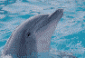# * and %x% operators in mxAlgebra

4 posts / 0 newOffline
Joined: 10/19/2009 - 15:38
* and %x% operators in mxAlgebra

anyone explain this?

> model2 <- mxModel(model1, mxAlgebra(.5*CTf, name="CTDZf"))
> model2 <- mxRun(model2)
Running SameMZDZf
Error in runHelper(model, frontendStart, intervals, silent, suppressWarnings, :
BLAS/LAPACK routine 'DPOTRF' gave error code -4

> model2 <- mxModel(model1, mxAlgebra(.5 %x% CTf, name="CTDZf"))
> model2 <- mxRun(model2)
Running SameMZDZf
Warning message:
In model 'SameMZDZf' NPSOL returned a non-zero status code 1. blah blah ...

yet
> mat <- matrix(rnorm(16), 4)
> .5 * mat == .5 %x% mat
[,1] [,2] [,3] [,4]
[1,] TRUE TRUE TRUE TRUE
[2,] TRUE TRUE TRUE TRUE
[3,] TRUE TRUE TRUE TRUE
[4,] TRUE TRUE TRUE TRUE

suspect something simple. if not, i can send the MxModel.
gregOffline
Joined: 07/31/2009 - 15:24
Which version of OpenMx are

Which version of OpenMx are you running? I get an error message under v. 1.0.4:

foo <- mxMatrix('Full', 2, 2, values = c(1:4), name = 'foo')
bar <- mxAlgebra(5 * foo, name = 'bar')
model <- mxModel('model', foo, bar)
out <- mxRun(model)
Error: The algebra 'bar' in model 'model' generated the error message: non-conformable arrays

There are three multiplication operators in OpenMx (operators and functions). "" performs elementwise-multiplication. "%%" performs matrix-multiplication. And "%x%" is the kronecker product. The reason for these three operators is that OpenMx is a matrix processing language. In R, the "*" operator will perform a element-scalar multiplication if one argument is scalar, or elementwise-multiplication if both arguments are matrices. In OpenMx, the scalar value is transformed into a 1 x 1 matrix.

In the OpenMx 1.1 release, the expression mxEval(bar, model, compute=TRUE) will return an error. It currently does not return an error, but this is a bug. We had to rewrite a significant portion of the evaluation routines in order to clean this up.Offline
Joined: 10/19/2009 - 15:38
merci, mike am using version

merci, mike

am using version 1.0.3-1505.

suggest that you change the documentation for mxAlgebra from

expression An R expression of matrix operators and matrix functions.

to

expression An expression of OpenMx-supported R matrix operators and matrix functions

"" is a valid matrix operator in R and in other matrix algebra languages because a 1 x 1 matrix is, by definition, a scalar and scalarmatrix is a legitimate operation.Offline
Joined: 07/31/2009 - 14:25
> expression An expression

> expression An expression of OpenMx-supported R matrix operators and matrix functions

done